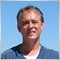# assignment to an array21

I'm trying to assign as follows (without using copyarray):

array1[i]=array2[j];

or

array1[i]=array2[j]+array2[k];

where i,j and k are integers, array1 and array2 are declared as double, and array2 contains values different from 0.
Somehow array1 remains 0 after the assignment.

Where did I go wrong?Moderator
13386

Please show sample of code. 1 line is not sample21

stringo wrote:
Please show sample of code. 1 line is not sample
Hope it will clarify the idea:

void example()
{
double a1[],a2[];
int i;

ArrayCopyRates(a1,"EURUSD", PERIOD_H1); // this line works fine

for (i=0;i<=100,i++)
a2[i]=a1[101-i]+a2[i]/5; // this is totally hypothetical but return zeros to a2
}Moderator
13386

First dimension of array must be declared explicitly

`double a1[],a2;`21

You were right. Thank you!